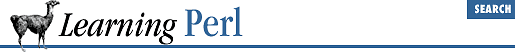home | O'Reilly's CD bookshelfs | FreeBSD | Linux | Cisco | Cisco Exam

### A.10 Chapter 11, Formats

1. Here's one way to do it:

```open(PW,"/etc/passwd") || die "How did you get logged in?";
while (<PW>) {
(\$user,\$uid,\$gcos) =

(split /

:/)[0,2,4];
(\$real) = split /,/,\$gcos;
write;
}

format STDOUT =
@<<<<<<< @>>>>>> @<<<<<<<<<<<<<<<<<<<<<<<<<<<<<<
\$user, \$uid, \$real
.```

The first line opens the password file. The ``` while``` loop processes the password file line by line. Each line is torn apart (with colon delimiters), loading up the scalar variables. The real name of the user is pulled out of the GCOS field. The final statement of the ``` while``` loop invokes ``` write``` to display all of the data.

The format for the ``` STDOUT``` filehandle defines a simple line with three fields. The values come from the three scalar variables that are given values in the ``` while``` loop.

2. Here's one way to do it:

```# append to program from the first problem...
format STDOUT_TOP =
======== ======= =========
.```

All it takes to get page headers for the previous program is to add a top-of-page format. Here, we put column headers on the columns.

To get the columns to line up, we copied the text of format ``` STDOUT``` and used overstrike mode in our text editor to replace ``` @<<<``` fields with ``` ====``` bars. That's the nice thing about the one-character-to-one-character correspondence between a format and the resulting display.

3. Here's one way to do it:

```# append to program from the first problem...
format STDOUT_TOP =
Page @<<<
\$%

Well, here again, to get stuff at the top of the page, I've added a top-of-page format. This format also contains a reference to ``` \$%``` , which gives me a page number automatically.A.9 Chapter 10, Filehandles and File TestsA.11 Chapter 12, Directory Access